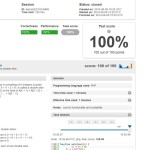# MaxDoubleSliceSum Demo Task at Codility.comCodility.com Tests 100/100 Solutions

Lesson 7 – Maximum slice problem – MaxDoubleSliceSum demo task solution code written in php. Gives 100/100 score at the time of publishing.

Solution in php:

```function solution(\$A) {
\$i = \$n = 0;
\$n = count(\$A);

if(3 == \$n){
return 0;
}

\$max_sum_end   = array_fill(0, \$n, 0);
\$max_sum_start = array_fill(0, \$n, 0);

for(\$i = 1; \$i < (\$n - 1); \$i++){
\$max_sum_end[\$i] = max(0, \$max_sum_end[\$i - 1] + \$A[\$i]);
}

for(\$i = \$n - 2; \$i > 0; \$i--){
\$max_sum_start[\$i] = max(0, \$max_sum_start[\$i + 1] + \$A[\$i]);
}

\$temp     = \$maxvalue = 0;

for(\$i = 1; \$i < (\$n - 1); \$i++){
\$temp = \$max_sum_end[\$i - 1] + \$max_sum_start[\$i + 1];
if(\$temp > \$maxvalue){
\$maxvalue = \$temp;
}
}

return \$maxvalue;
}
```

Given “AS IS”, can be ported from other languages from solutions found on the internet, please use with care.

Please note: we think that codility.com does not give a correct assessment of your real-world programming skills. For instance, reading the below would bring more understanding why: //x20x.co.uk/2014/02/why-i-refuse-to-use-codility-and-so-should-you/

This site uses Akismet to reduce spam. Learn how your comment data is processed.